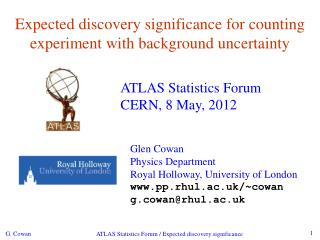Download PresentationExpected discovery significance for counting experiment with background uncertainty

# Expected discovery significance for counting experiment with background uncertainty - PowerPoint PPT PresentationDownload Presentation## Expected discovery significance for counting experiment with background uncertainty

- - - - - - - - - - - - - - - - - - - - - - - - - - - E N D - - - - - - - - - - - - - - - - - - - - - - - - - - -
##### Presentation Transcript

1. Expected discovery significance for counting experiment with background uncertainty ATLAS Statistics Forum CERN, 8 May, 2012 Glen Cowan Physics Department Royal Holloway, University of London www.pp.rhul.ac.uk/~cowan g.cowan@rhul.ac.uk ATLAS Statistics Forum / Expected discovery significance

2. Outline / executive summary I. Discovery sensitivity for counting experiment with b known: (a) (b) Profile likelihood ratio test & Asimov: II. Discovery sensitivity with uncertainty in b, σb: (a) (b) Profile likelihood ratio test & Asimov: ATLAS Statistics Forum / Expected discovery significance

3. Counting experiment with known background Count a number of events n ~ Poisson(s+b), where s = expected number of events from signal, b = expected number of background events. To test for discovery of signal compute p-value of s=0 hypothesis, Usually convert to equivalent significance: where Φ is the standard Gaussian cumulative distribution, e.g., Z > 5 (a 5 sigma effect) means p < 2.9 ×10-7. To characterize sensitivity to discovery, give expected (mean or median) Z under assumption of a given s. ATLAS Statistics Forum / Expected discovery significance

4. s/√b for expected discovery significance For large s + b, n → x ~ Gaussian(m,s) , m = s + b, s = √(s + b). For observed value xobs, p-value of s = 0 is Prob(x > xobs | s = 0),: Significance for rejecting s = 0 is therefore Expected (median) significance assuming signal rate s is ATLAS Statistics Forum / Expected discovery significance

5. Better approximation for significance Poisson likelihood for parameter s is For now no nuisance params. To test for discovery use profile likelihood ratio: So the likelihood ratio statistic for testing s = 0 is ATLAS Statistics Forum / Expected discovery significance

6. Approximate Poisson significance (continued) For sufficiently large s + b, (use Wilks’ theorem), To find median[Z|s], let n → s + b (i.e., the Asimov data set): This reduces to s/√b for s << b. ATLAS Statistics Forum / Expected discovery significance

7. n ~ Poisson(s+b), median significance,assuming s, of the hypothesis s = 0 CCGV, EPJC 71 (2011) 1554, arXiv:1007.1727 “Exact” values from MC, jumps due to discrete data. Asimov √q0,A good approx. for broad range of s, b. s/√b only good for s « b. ATLAS Statistics Forum / Expected discovery significance

8. Extending s/√b to case where b uncertain The intuitive explanation of s/√b is that it compares the signal, s, to the standard deviation of n assuming no signal, √b. Now suppose the value of b is uncertain, characterized by a standard deviation σb. A reasonable guess is to replace √b by the quadratic sum of √b and σb, i.e., This has been used to optimize some analyses e.g. where σb cannot be neglected. ATLAS Statistics Forum / Expected discovery significance

9. Profile likelihood with b uncertain This is the well studied “on/off” problem: Cranmer 2005; Cousins, Linnemann, and Tucker 2008; Li and Ma 1983,... Measure two Poisson distributed values: n ~ Poisson(s+b) (primary or “search” measurement) m ~ Poisson(τb) (control measurement, τ known) The likelihood function is Use this to construct profile likelihood ratio (b is nuisance parmeter): ATLAS Statistics Forum / Expected discovery significance

10. Asymptotic significance Use profile likelihood ratio for q0, and then from this get discovery significance using asymptotic approximation (Wilks’ theorem): Essentially same as in: ATLAS Statistics Forum / Expected discovery significance

11. Asimov approximation for median significance To get median discovery significance, replace n, m by their expectation values assuming background-plus-signal model: n → s + b m → τb ˆ Or use the variance of b = m/τ, , to eliminate τ: ATLAS Statistics Forum / Expected discovery significance

12. Limiting cases Expanding the Asimov formula in powers of s/b and σb2/b (= 1/τ)gives So the “intuitive” formula can be justified as a limiting case of the significance from the profile likelihood ratio test evaluated with the Asimov data set. ATLAS Statistics Forum / Expected discovery significance

13. Testing the formulae: s = 2 Structure in points (MC) is due to discreteness of data. ATLAS Statistics Forum / Expected discovery significance

14. Testing the formulae: s = 5 ATLAS Statistics Forum / Expected discovery significance

15. Testing the formulae: s = 10 ATLAS Statistics Forum / Expected discovery significance

16. Summary Simple formula for expected discovery significance based on profile likelihood ratio test and Asimov approximation: For details see accompanying note on agenda page or www.pp.rhul.ac.uk/~cowan/stat/medsig/medsigNote.pdf For large b, all formulae OK. For small b, s/√b and s/√(b+σb2) overestimate the significance. Could be important in optimization of searches with low background. Formula maybe also OK if model is not simple on/off experiment, e.g., several background control measurements (check this). ATLAS Statistics Forum / Expected discovery significance

17. Extra slides ATLAS Statistics Forum / Expected discovery significance

18. Ingredients for profile likelihood ratio To construct the profile likelihood ratio we need the estimators: and in particular to test for discovery (s = 0), ATLAS Statistics Forum / Expected discovery significance